# Greater Than Sign – Definition with Examples

## Introduction

Inequality between two values, quantities, or numbers, where one is more than the others is what we mean by greater than in Math. In other words, when A is more in comparison to B, we express it as A is greater than B.

## What Is the Symbol of Greater Than?

The symbol of greater than is ‘>’. Let us understand greater than sign with examples given below:

The symbol looks like an alligator’s mouth open in front of the bigger number or quantity.

The alligator method is the best way to help young learners retain the usage of the more than sign. To enable them to have a firm grip over the concept, parents and teachers can indulge in fun activities like creating its symbol in an alligator’s mouth and have kids place them between two quantities shown to them.

Another concept used in this regard is less-than. Less than also compares two values, quantities, or numbers. The difference is that here, one is less than the other. As per the above example, where A is more than B, we can also say that B is less than A. The symbol of less than is <.

Let us take part in a short activity to understand the greater than and less than signs

Step 1: Create a mouth with your left and right hands, as shown below.

Step 2: Observe one hand at a time.

The right hand creates a greater-than sign while the left hand creates a less-than sign. If you ever get confused, remember that your right hand is the greater-than alligator, and your left hand is the less-than alligator.

For example, the teacher displays a big box of candies on one side, a handful on the other, and then asks a student to place the greater-than sign between the two displayed quantities. Participating in such activities can interest your ward more than mundane worksheets, facilitating better learning.

Parents and guardians can come up with other such activities to interest their young learners and help them grasp concepts better. Using everyday objects to compare their quantities is also a great way to impart the basics of the concept of greater and less.

## Solved Examples

Let us look at some of these solved examples to understand them better.

Example 1: Use the correct sign (< , >) in the following.

i.

Solution: <

< is the correct sign because 5 is greater than 4, and the open mouth faces the bigger number. Thus, the open mouth faces the side with 5 stars, and the closed end faces the smaller number.

ii.

Solution: >

> is the correct sign because 6 is greater than 5, and the open mouth faces the bigger number. Thus, the open mouth of the symbol faces the 6 pairs of cherries, which is greater than the side with 5 pairs of cherries.

iii.

Solution: <

< is the correct sign because 8 is greater than 6 and the open mouth of the symbol faces the side with the bigger number. Thus, the open mouth faces the side with 8 suns since the other side has fewer suns.

Example 2: Use the correct sign (< , >) in the following.

Solution: <

< is the correct sign because 6 is greater than 4 and the open mouth of the symbol faces the bigger number. Thus, the open mouth faces the side with 6 lollipops since the other side has fewer lollipops.

Example 3: Draw the alligator’s mouth facing the bigger number.

i.

ii.

Solution:

i. >

ii. <

Since the mouth of the alligator opens toward the bigger number, the answer to (i) is > because 15 is > 11, and the answer to (ii) is < because 64 is greater than 46.

## Practice Problems

It is brainstorm o’clock, Junior!

1

### Choose the correct sign: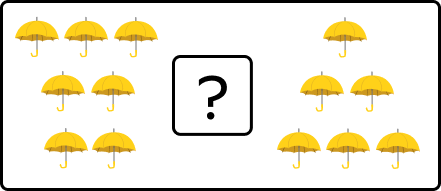$>$
$\lt$
$=$
None of the above
CorrectIncorrect
Correct answer is: $>$
> is the correct sign because 7 is greater than 6 and the open mouth of the symbol faces the bigger number. Thus, the open mouth faces the side with 7 umbrellas since the other side has fewer umbrellas.
2

### Choose the correct sign: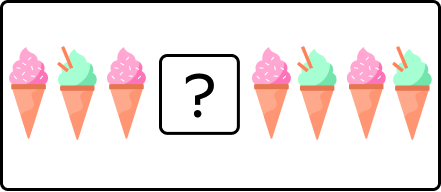$\lt$
$\gt$
=
None of the above
CorrectIncorrect
Correct answer is: $\lt$
$\lt$ is the correct sign because 4 is greater than 3 and the open mouth of the symbol faces the bigger number. Thus, the open mouth faces the side with 4 ice creams since the other side has fewer ice creams.
3

### Draw the alligator mouth correctly.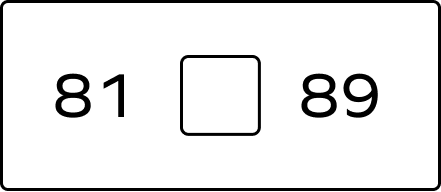$\lt$
$\gt$
None of the above
=
CorrectIncorrect
Correct answer is: $\lt$
81 is less than 89
4

### Draw the alligator mouth correctly.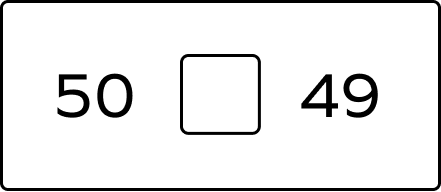$\lt$
$\gt$
None of the above
=
CorrectIncorrect
Correct answer is: $\gt$
50 is greater than 49
5

### Draw the alligator mouth correctly.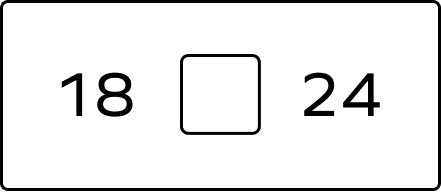$\lt$
$\gt$
None of the above
=
CorrectIncorrect
Correct answer is: $\lt$
The mouth of the alligator opens towards the bigger number.
Thus, it opens towards 89 since 89 $\gt$ 81; it opens towards 50 since 50 $\gt$ 49; it opens towards 24 since 24 $\gt$ 18.
6

### Is the following true?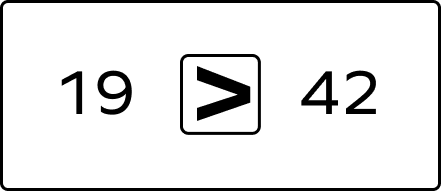Yes
No
CorrectIncorrect
Since 42 $>$ 19, the open mouth should face 42.